Welcome GuestYou last visited September 25, 2020, 1:54 am
All times shown are
Eastern Time (GMT-5:00)

# Florida man wins multimillion lottery jackpot for 2nd time

Topic closed. 71 replies. Last post 7 years ago by jamella724.

 Page 4 of 5
 Jon DLos Angeles, CaliforniaUnited StatesMember #103809January 5, 20111530 PostsOffline November 2, 2013, 7:32 pm⮟Quote: Originally posted by Stack47 on November 2, 2013"That's not "life changing" but mainly privides security for himself and his loved ones."I never saw where winning a life changing jackpot means a person must change their lifestyle. It's obvious Mr. Bozeman enjoyed playing the Lotto game; where is it written he must quit because he won a jackpot.There is nothing wrong with the "I would quit" opinions either, but there is a big difference because Bozeman is speaking from a real experience.Yeah, we only sometimes hear about the ones that win a few million and think they can party like Kim Kardashian in Paris and Dubai, buying mansions and Lambos and went broke in a few years.The smart ones keep it real, stay grounded. Seems like James B. has it under control.Hope he wins a 3rd JP with his 3rd set of numbers, that would really be something!
 larry3100Redwood City,CaliforniaUnited StatesMember #70501February 3, 2009211 PostsOffline November 3, 2013, 6:26 pm There's no such thing as lucky lottery numbers! Just pure luck, that's all.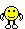'
 BuyLowFloridaUnited StatesMember #61433May 22, 20081211 PostsOffline November 4, 2013, 11:16 pmThe self pick numbers he won with are below, anyone see any patterns?? 8/31/13 -- 10, 11, 12, 17, 28, 46 6/16/12 -- 5, 7, 15, 30, 46, 48
 helpmewin100u\$aUnited StatesMember #106661February 22, 201119967 PostsOffline November 4, 2013, 11:26 pmQuote: Originally posted by BuyLow on November 4, 2013The self pick numbers he won with are below, anyone see any patterns?? 8/31/13 -- 10, 11, 12, 17, 28, 46 6/16/12 -- 5, 7, 15, 30, 46, 48Thanks BuyLowi'm gonna make 7-11 my favorite store when i'm in florida sounds like a Lucky store to buy tickets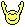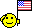BuyLowFloridaUnited StatesMember #61433May 22, 20081211 PostsOffline November 5, 2013, 6:09 amQuote: Originally posted by helpmewin on November 4, 2013Thanks BuyLowi'm gonna make 7-11 my favorite store when i'm in florida sounds like a Lucky store to buy tickets7-11 is ok, but Publix supermarkets are the hands down winner of selling winning tickets.  I'm guessing only because so many people buy there tickets there.
 psykomoUnited StatesMember #4877May 30, 20045289 PostsOffline November 5, 2013, 6:20 amQuote: Originally posted by BuyLow on November 4, 2013The self pick numbers he won with are below, anyone see any patterns?? 8/31/13 -- 10, 11, 12, 17, 28, 46 6/16/12 -- 5, 7, 15, 30, 46, 48BL:THEY R BIRTHDAY NUMBER\$\$\$\$\$\$\$\$\$VERY GOOD ONE\$\$\$\$\$\$\$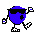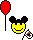PLAYED wives # 6/16/12>>>>>>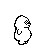WON GOOD ONE\$\$\$\$\$\$\$PLAY IT again BOZ use UR 46 year10-11-12-17-28-46 MOE\$\$\$\$\$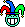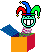rdgrnr100Way back up in them dadgum hills, son!United StatesMember #73902April 28, 200914903 PostsOffline November 5, 2013, 9:17 amQuote: Originally posted by BuyLow on November 4, 2013The self pick numbers he won with are below, anyone see any patterns?? 8/31/13 -- 10, 11, 12, 17, 28, 46 6/16/12 -- 5, 7, 15, 30, 46, 48"The self pick numbers he won with are below, anyone see any patterns??"Ah, you knew I just couldn't resist a challenge like that, didn't you? Well, I think this is as close to his formula as we'll be able to get based on my calculations. I may, however, be off a degree or two on the ramaframastan quotient:  First off, his picks have a pair of numbers, eg 10 and 48. Ciclometrica The distance between the two is given by their difference, ie 86-70 = 16. If we denote the two numbers respectively with the letters "b"and"c"distance can be expressed algebraically as"CB". Adding the item right away you get a shift symmetric time:  70 (16) 86 (+16) is 12Generalizing the operation carried out algebraically, indicating with "d"the number 12, we have:d = c + (cb) => d = 2c-b d = 2 x 86 to 70 = 172-70 = 102 (out of 90) = 12Since the three numbers on the circumference ciclometrica form an equilateral triangle the number 12 is called "triangular closure." However, we have another chance to close a triangular symmetrical by moving counterclockwise.  54? (-16) 70 (16) 86 (16)This time, to derive the closure counter-clockwise "to"we must subtract from the left side"b" distance "cb":a = b - (cb) => a = 2b-c  a = 2 x 70 - 86 = 140-86 = 54If the basic numbers are marked with the letters "b"and"c", the two closures are obtained with the following triangular formulette:d = 2c-b,            a = 2b-c; The isosceles triangle expresses the symmetry in ciclometria easier.In order to have available at a glance all the symmetries between one or more pairs of numbers the Manna planted a simple statement, called ciclodinamiche before then, more appropriately, "symmetry":   2a  2b   -a  -  2b-a   -b  2a-b  - On the flat line you write numbers double base, while the first column on the left are reported complementary to 90 base numbers. In inner cells, as in a multiplication table, we will cross the amounts of external values. Compile the prospectus for the pair considered 70 and 86.For a = 70 b = 86, these are the values to be inserted on the outside line:  2nd = 2 x 70 = 50,                  2b = 2 x 86 = 82;-A = 90 - 70 = 20,                  b-= 90 - 86 = 4;Omitting the sums of external values reported in the same letter, as you regain the starting numbers, here is the prospectus with the bilateral symmetry of the pair 70-86:   50  82   20  -  12   4  54  - The problem is then proposed to deal with Manna was to be able to go back to basic numbers, generators of symmetry, the projections being known only bilateral (or triangular closure), that the internal values of the prospectus.Let us assume that the internal numbers are known only, that is, the bilateral symmetry 12 and 54. As you climb to the torque-generating? We assign to the uncertainties of the letters "a" and "b", while describing the terms known, 12 and 54, respectively, with the letters A and B. Knowing that A = (2b-a) and B = (2a-b), to determine "a" and "b" is enough to solve a simple system of linear equations:A = 2b-a                    B = 2a-bWe get "a" from the first equation and substitute the result in the second:a = 2b-A,                    B = 2 (2b - A) - B isB = 3b - 2ASince the last equation we obtain the unknown "b":b = (2A + B): 3 Substituting the values of "b" in the first equation we can also find the unknown "to":a = 2b-2 A = ((2A + B): 3)-A = (4A +2 B) :3-A is a = (2B + A): 3This is the formula for DIEMME two numbers. We should add, however, some detail. Since the denominator is the number 3 has to result in a whole number is necessary that the numerator is divisible by 3: the precondition is that the numbers A and B belong to the same triple figures.Now verify the formula for the pair Diemme Example 12-54:a = (2B + A) 3 = (2 x 54 +12): 3 = (108 +12): 3 = 120:3 =40b = (2A + B): 3 = (2 x 12 +54): 3 = (24 +54): 3 = 78:3 =26The numbers 26 and 40, then, are those who through bilateral projection of the distance generate the pair 54-12. But the initial numbers were not 70 and maybe 86? Indeed they are! The numbers that we were at first considered this, but we have not committed any mistake. Using Diemme we divided the numbers by 3 and arithmetic ciclometrico clock when we divide a number by 3 we consider three modular valid: the three terms of the same triplet symmetric distance 30. These are good solutions:a = 120: 3 = 40or 10or 70;b = 78 3 = 26or 56or 86.The third part of a number is equal to an entire triplet symmetric distance 30, that is an equilateral triangle. In fact, if we try to triple the elements that form a ciclometria figures above, for example 13-43-73, we get the same result:3 x 13 = 39,            3 x 43 = 129 = 39,            3 x 73 = 219 = 39.Now implanting the statement of symmetries for a set of three numbers abc:    2a  2b  2c   -a  -  2b-a  2c-a   -b  2a-b  -  2c-b   -c  2a-c  2b-c  - We need to find relationships that allow us to go back to the original triad abc knowing the following interior elements:A = 2c-a,    B = 2a-b,    C = 2b-c.The solution, which is easily obtained by solving a system of equations with three unknowns, is as follows:a = (A + 4B +2 C) 7                        b = (4C +2 A + B) 7                        c = (4A +2 B + C): 7This is the formula Diemme, in pure form, on three numbers. In the denominator we have the number 7 and this complicates a little 'things, because it is not easy to trace the triads that make the numerator modularly divisible by this number. But the arithmetic of forms and of the remains comes to our aid. In mathematics, dividing by a number "n" means multiply by its reciprocal (1 / n). Lot of arithmetic, to form 90, the reciprocal of 1 / 7 is 13. In practice, divide by 7 means multiply by 13, and vice versa. The three formulas are thus converted into Diemme:a = (4B +2 C + A) x 13                  b = (4C +2 A + B) x 13                  c = (4A +2 B + C) x 13 The formula used to calculate Diemme formations through which the game will generate the symmetries of the other, but nothing prevents us (we'll do it later) to determine a priori which features must have the training source.Extraction No. 44 of '03 on June 3, 2004 Torino wheel diameter can trace both the 9-54, Sum 63. The additional amount of 63 to 91 is 28, numbers that track on Palermo.We form two columns of three numbers, inserting the two diametrical, alternatively, the 1st and 3rd place. A  9  a      A  54  a   B  28    b      B  28  b   C  54  c      C  9  c We have to apply DIEMME to determine the three elements of the two triplets of origin, but you can do without the three formulas using a fast numerical technique, always sensed by Manna, called LINE OF SYMMETRY.Take the first triplet, where A = 9, B and C = 28 = 54. Using the formulas we get:a = (A + 4B + 2C) x 13 = (9 + 28 + 4 x 2 x 54) x 13 = 2977 = 7 b = (2A + B + 4C) x 13 = (2 x 4 x 9 + 28 + 54) x 13 = 3406 = 76 c = (4A + 2B + C) x 13 = (4 x 9 + 2 x 28 + 54) x13 = 1898 = 8 The three numbers 7-76-8, projecting symmetrically training 9-28-54 note, however, can be calculated in a way much more quickly. Calculated the "c", the foot of the column with the formula c = 52A +26 B +13 C,we can go back to the other two unknowns with simple calculations. A  9    a   B  28    b   C  54  8  c Multiply by two the "c" and subtract the value of A: 2 x 8-9 = 16-9 = 7.So, double the number found "a", 7, and subtract B: 2 x 7 - 28 = 14-28 = 76. A  9  7  a  2 x 8-9   B  28  76  b  2 x 7-28   C  54  8  c   Calculated on the base of the formation incognita, using the chain of symmetry we were able to reassemble it in its entirety. Proceeding similarly for the second triad we obtain: A  54  52  a  2 x 53-54   B  28  76  b  2 x 52-28   C  9  53  c   We got two new triplets logically add up to 91. For both the middle number is 76, we drop the prediction. The other four numbers make up the rectangle 7-8-52-53, at stake for both the wheels of Palermo and Turin for a dozen hits (writing after the draw No. 46, June 9).Note: 6th shot 7-53 on both Turin Palermo 8 th hit on 8-53And then double it.
 RJOh100mid-OhioUnited StatesMember #9March 24, 200120272 PostsOffline November 5, 2013, 9:36 amQuote: Originally posted by BuyLow on November 4, 2013The self pick numbers he won with are below, anyone see any patterns?? 8/31/13 -- 10, 11, 12, 17, 28, 46 6/16/12 -- 5, 7, 15, 30, 46, 48I suspect if he is using a system to pick his numbers, it's dynamic and the variable he used can't be discovered by looking at the results, it could be something as simple as the numbers in the previous drawing.What ever he was doing, he didn't mind doing it for another year before getting another jackpot winner.  Even a winning system takes time to score big. * you don't need to buy every combination, just the winning ones *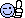helpmewin100u\$aUnited StatesMember #106661February 22, 201119967 PostsOffline November 5, 2013, 10:09 amQuote: Originally posted by 1977 on November 1, 2013Well he won on June 16,2012  with his self pick numbers and Aug.31,2013--with his self pick numbers----15 months apart---------mmmmmmm!!!! Happy for him....BUT I don't think I would play after the first \$10million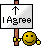he's 67-year-old maybe he plays the lottery just for a hobby what else does he have to do.faber98United StatesMember #108376March 25, 2011505 PostsOffline November 5, 2013, 10:10 amQuote: Originally posted by jjtheprince on November 1, 2013He should buy MM tickets for tonight and PB tickets for tomorrow night.  If he did, I bet he would win both drawings.no, he shouldn't. he won easier to hit state lottery for a paltry 10m and 3m. 90% of the people on here sneer at state lotteries because they "want" to play the big ones that they can never hit. nothing wrong with winning under 10m. no one can handle the problems with winning massive amounts of money that you are all chasing.
 faber98United StatesMember #108376March 25, 2011505 PostsOffline November 5, 2013, 10:15 amQuote: Originally posted by RedStang on November 1, 2013Most people would quit the first time they won, but it seems like this guy just likes to play lotto. Curious to know how many sets of numbers he plays everytime.after winning the first one, why stop ? certainly he can afford to play every state lottery drawing and those nasty big ones too. it paid off for him. no reason to stop playing just because you won once. no such thing as the "law of averages" meaning if you win once you can't win twice or more.
 helpmewin100u\$aUnited StatesMember #106661February 22, 201119967 PostsOffline November 5, 2013, 10:21 amQuote: Originally posted by rdgrnr on November 5, 2013"The self pick numbers he won with are below, anyone see any patterns??"Ah, you knew I just couldn't resist a challenge like that, didn't you? Well, I think this is as close to his formula as we'll be able to get based on my calculations. I may, however, be off a degree or two on the ramaframastan quotient:  First off, his picks have a pair of numbers, eg 10 and 48. Ciclometrica The distance between the two is given by their difference, ie 86-70 = 16. If we denote the two numbers respectively with the letters "b"and"c"distance can be expressed algebraically as"CB". Adding the item right away you get a shift symmetric time:  70 (16) 86 (+16) is 12Generalizing the operation carried out algebraically, indicating with "d"the number 12, we have:d = c + (cb) => d = 2c-b d = 2 x 86 to 70 = 172-70 = 102 (out of 90) = 12Since the three numbers on the circumference ciclometrica form an equilateral triangle the number 12 is called "triangular closure." However, we have another chance to close a triangular symmetrical by moving counterclockwise.  54? (-16) 70 (16) 86 (16)This time, to derive the closure counter-clockwise "to"we must subtract from the left side"b" distance "cb":a = b - (cb) => a = 2b-c  a = 2 x 70 - 86 = 140-86 = 54If the basic numbers are marked with the letters "b"and"c", the two closures are obtained with the following triangular formulette:d = 2c-b,            a = 2b-c; The isosceles triangle expresses the symmetry in ciclometria easier.In order to have available at a glance all the symmetries between one or more pairs of numbers the Manna planted a simple statement, called ciclodinamiche before then, more appropriately, "symmetry":   2a  2b   -a  -  2b-a   -b  2a-b  - On the flat line you write numbers double base, while the first column on the left are reported complementary to 90 base numbers. In inner cells, as in a multiplication table, we will cross the amounts of external values. Compile the prospectus for the pair considered 70 and 86.For a = 70 b = 86, these are the values to be inserted on the outside line:  2nd = 2 x 70 = 50,                  2b = 2 x 86 = 82;-A = 90 - 70 = 20,                  b-= 90 - 86 = 4;Omitting the sums of external values reported in the same letter, as you regain the starting numbers, here is the prospectus with the bilateral symmetry of the pair 70-86:   50  82   20  -  12   4  54  - The problem is then proposed to deal with Manna was to be able to go back to basic numbers, generators of symmetry, the projections being known only bilateral (or triangular closure), that the internal values of the prospectus.Let us assume that the internal numbers are known only, that is, the bilateral symmetry 12 and 54. As you climb to the torque-generating? We assign to the uncertainties of the letters "a" and "b", while describing the terms known, 12 and 54, respectively, with the letters A and B. Knowing that A = (2b-a) and B = (2a-b), to determine "a" and "b" is enough to solve a simple system of linear equations:A = 2b-a                    B = 2a-bWe get "a" from the first equation and substitute the result in the second:a = 2b-A,                    B = 2 (2b - A) - B isB = 3b - 2ASince the last equation we obtain the unknown "b":b = (2A + B): 3 Substituting the values of "b" in the first equation we can also find the unknown "to":a = 2b-2 A = ((2A + B): 3)-A = (4A +2 B) :3-A is a = (2B + A): 3This is the formula for DIEMME two numbers. We should add, however, some detail. Since the denominator is the number 3 has to result in a whole number is necessary that the numerator is divisible by 3: the precondition is that the numbers A and B belong to the same triple figures.Now verify the formula for the pair Diemme Example 12-54:a = (2B + A) 3 = (2 x 54 +12): 3 = (108 +12): 3 = 120:3 =40b = (2A + B): 3 = (2 x 12 +54): 3 = (24 +54): 3 = 78:3 =26The numbers 26 and 40, then, are those who through bilateral projection of the distance generate the pair 54-12. But the initial numbers were not 70 and maybe 86? Indeed they are! The numbers that we were at first considered this, but we have not committed any mistake. Using Diemme we divided the numbers by 3 and arithmetic ciclometrico clock when we divide a number by 3 we consider three modular valid: the three terms of the same triplet symmetric distance 30. These are good solutions:a = 120: 3 = 40or 10or 70;b = 78 3 = 26or 56or 86.The third part of a number is equal to an entire triplet symmetric distance 30, that is an equilateral triangle. In fact, if we try to triple the elements that form a ciclometria figures above, for example 13-43-73, we get the same result:3 x 13 = 39,            3 x 43 = 129 = 39,            3 x 73 = 219 = 39.Now implanting the statement of symmetries for a set of three numbers abc:    2a  2b  2c   -a  -  2b-a  2c-a   -b  2a-b  -  2c-b   -c  2a-c  2b-c  - We need to find relationships that allow us to go back to the original triad abc knowing the following interior elements:A = 2c-a,    B = 2a-b,    C = 2b-c.The solution, which is easily obtained by solving a system of equations with three unknowns, is as follows:a = (A + 4B +2 C) 7                        b = (4C +2 A + B) 7                        c = (4A +2 B + C): 7This is the formula Diemme, in pure form, on three numbers. In the denominator we have the number 7 and this complicates a little 'things, because it is not easy to trace the triads that make the numerator modularly divisible by this number. But the arithmetic of forms and of the remains comes to our aid. In mathematics, dividing by a number "n" means multiply by its reciprocal (1 / n). Lot of arithmetic, to form 90, the reciprocal of 1 / 7 is 13. In practice, divide by 7 means multiply by 13, and vice versa. The three formulas are thus converted into Diemme:a = (4B +2 C + A) x 13                  b = (4C +2 A + B) x 13                  c = (4A +2 B + C) x 13 The formula used to calculate Diemme formations through which the game will generate the symmetries of the other, but nothing prevents us (we'll do it later) to determine a priori which features must have the training source.Extraction No. 44 of '03 on June 3, 2004 Torino wheel diameter can trace both the 9-54, Sum 63. The additional amount of 63 to 91 is 28, numbers that track on Palermo.We form two columns of three numbers, inserting the two diametrical, alternatively, the 1st and 3rd place. A  9  a      A  54  a   B  28    b      B  28  b   C  54  c      C  9  c We have to apply DIEMME to determine the three elements of the two triplets of origin, but you can do without the three formulas using a fast numerical technique, always sensed by Manna, called LINE OF SYMMETRY.Take the first triplet, where A = 9, B and C = 28 = 54. Using the formulas we get:a = (A + 4B + 2C) x 13 = (9 + 28 + 4 x 2 x 54) x 13 = 2977 = 7 b = (2A + B + 4C) x 13 = (2 x 4 x 9 + 28 + 54) x 13 = 3406 = 76 c = (4A + 2B + C) x 13 = (4 x 9 + 2 x 28 + 54) x13 = 1898 = 8 The three numbers 7-76-8, projecting symmetrically training 9-28-54 note, however, can be calculated in a way much more quickly. Calculated the "c", the foot of the column with the formula c = 52A +26 B +13 C,we can go back to the other two unknowns with simple calculations. A  9    a   B  28    b   C  54  8  c Multiply by two the "c" and subtract the value of A: 2 x 8-9 = 16-9 = 7.So, double the number found "a", 7, and subtract B: 2 x 7 - 28 = 14-28 = 76. A  9  7  a  2 x 8-9   B  28  76  b  2 x 7-28   C  54  8  c   Calculated on the base of the formation incognita, using the chain of symmetry we were able to reassemble it in its entirety. Proceeding similarly for the second triad we obtain: A  54  52  a  2 x 53-54   B  28  76  b  2 x 52-28   C  9  53  c   We got two new triplets logically add up to 91. For both the middle number is 76, we drop the prediction. The other four numbers make up the rectangle 7-8-52-53, at stake for both the wheels of Palermo and Turin for a dozen hits (writing after the draw No. 46, June 9).Note: 6th shot 7-53 on both Turin Palermo 8 th hit on 8-53And then double it.WOW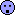you sure know your stuff rdgrnrhelpmewin100u\$aUnited StatesMember #106661February 22, 201119967 PostsOffline November 5, 2013, 10:24 amQuote: Originally posted by BuyLow on November 5, 20137-11 is ok, but Publix supermarkets are the hands down winner of selling winning tickets.  I'm guessing only because so many people buy there tickets there.yes your right ticket sales has a big play in it, either one will be goodRJOh100mid-OhioUnited StatesMember #9March 24, 200120272 PostsOffline November 5, 2013, 1:05 pmQuote: Originally posted by faber98 on November 5, 2013no, he shouldn't. he won easier to hit state lottery for a paltry 10m and 3m. 90% of the people on here sneer at state lotteries because they "want" to play the big ones that they can never hit. nothing wrong with winning under 10m. no one can handle the problems with winning massive amounts of money that you are all chasing.No all state games have jackpot of paltry amounts.  Ohio's Classic Lotto(6/49) has a \$50.6M jackpot and its Rolling Cash5(5/39) game rolled to \$598K last night.  I'm certainly not one of those LP members who sneering at those amounts. * you don't need to buy every combination, just the winning ones *BuyLowFloridaUnited StatesMember #61433May 22, 20081211 PostsOffline November 5, 2013, 1:23 pmQuote: Originally posted by RJOh on November 5, 2013No all state games have jackpot of paltry amounts.  Ohio's Classic Lotto(6/49) has a \$50.6M jackpot and its Rolling Cash5(5/39) game rolled to \$598K last night.  I'm certainly not one of those LP members who sneering at those amounts.I sneer at no amount, they are all welcomed and I am grateful for them.  A couple more zeros is obviously better lol.....

 Page 4 of 5